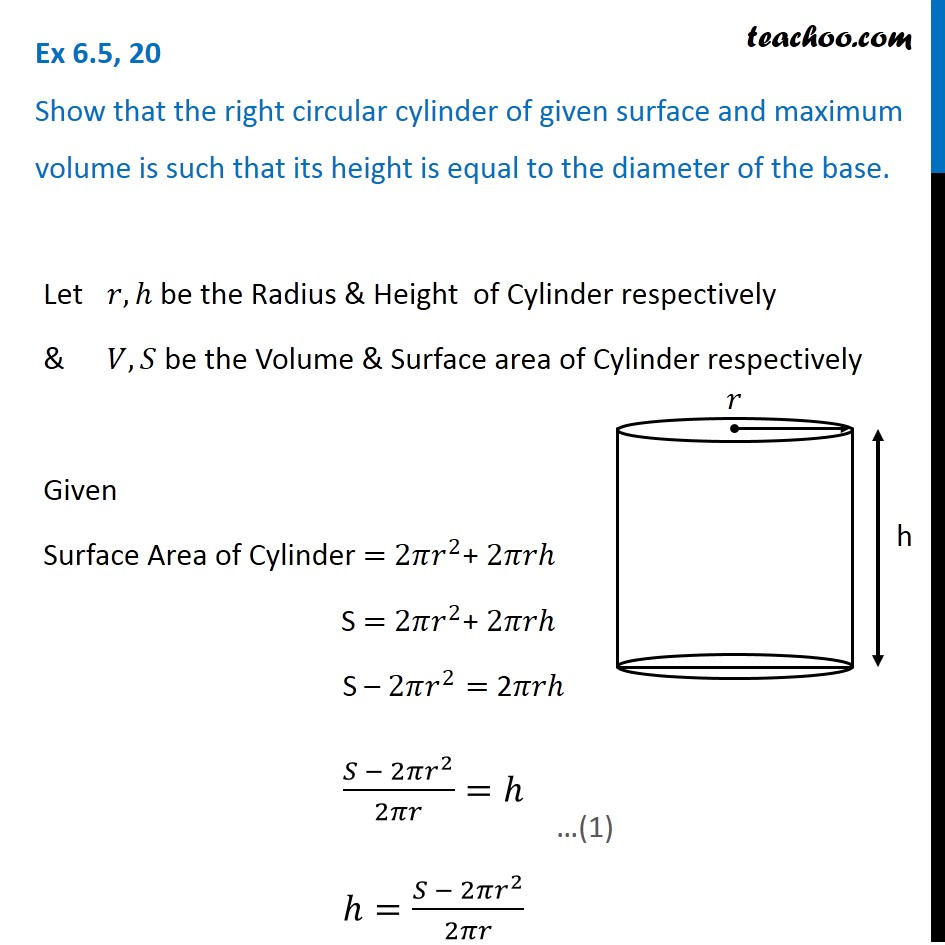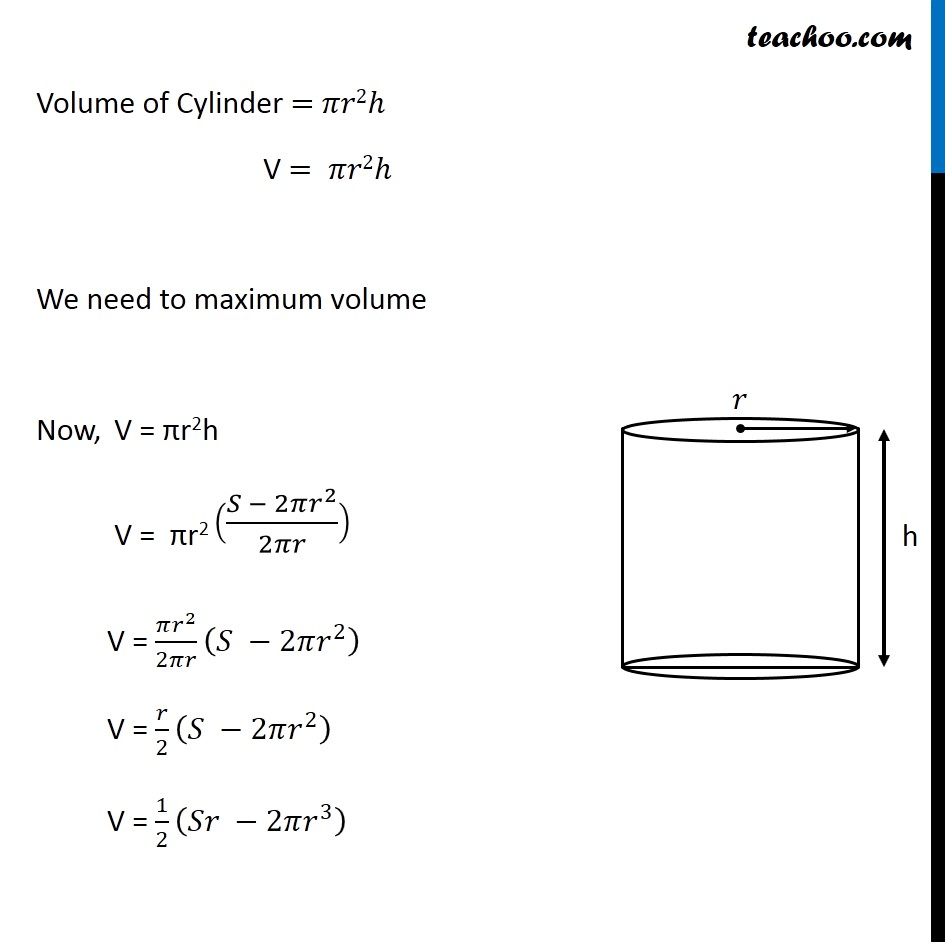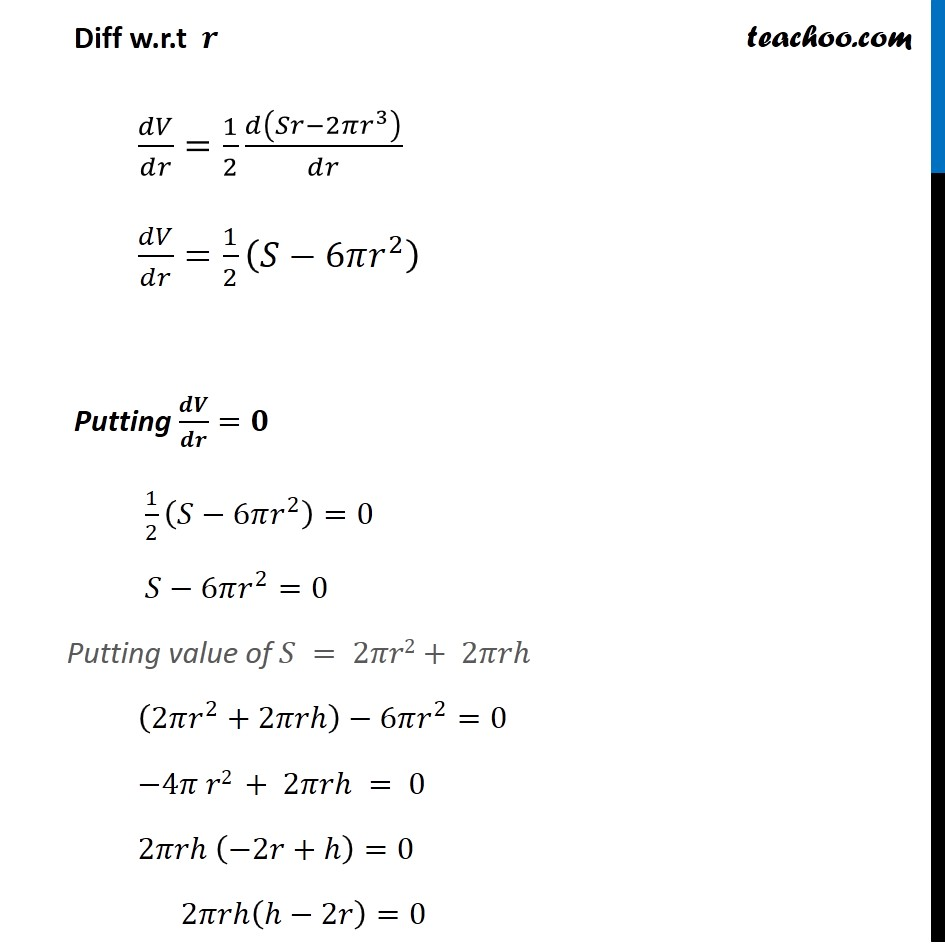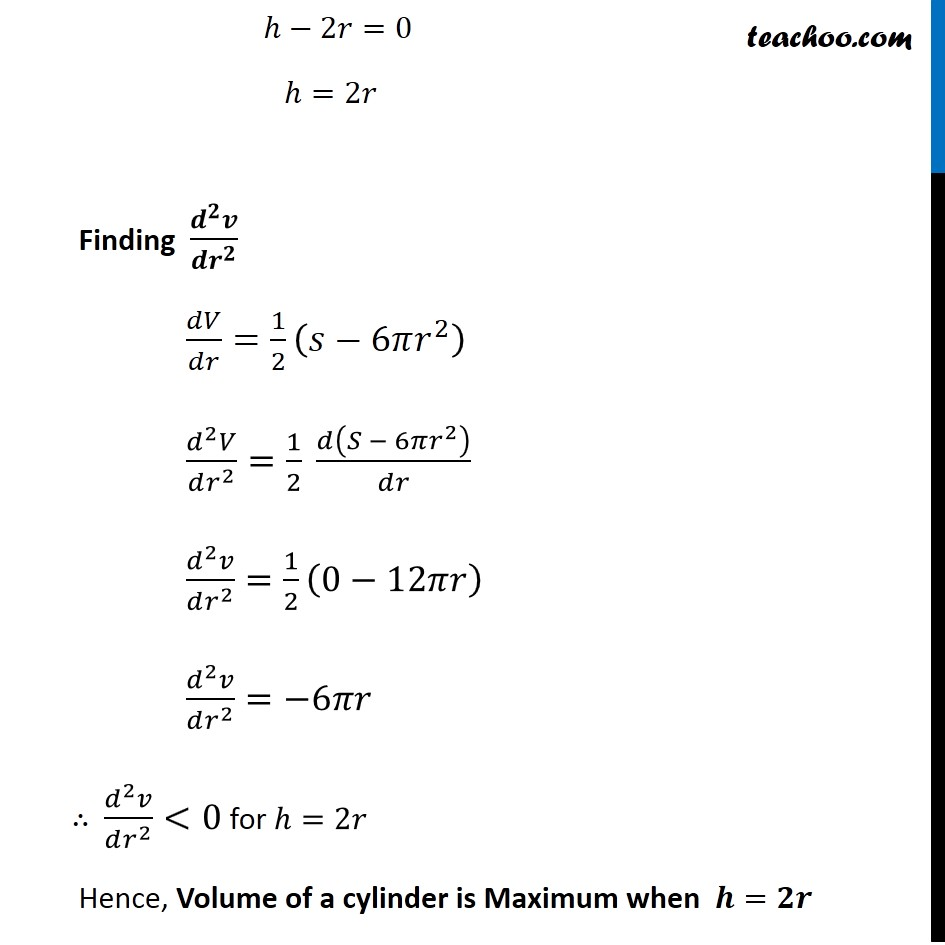Minima/ maxima (statement questions) - Geometry questions

Chapter 6 Class 12 Application of Derivatives
Concept wiseIntroducing your new favourite teacher - Teachoo Black, at only ₹83 per month

### Transcript

Ex 6.5, 20 Show that the right circular cylinder of given surface and maximum volume is such that its height is equal to the diameter of the base. Let 𝑟, ℎ be the Radius & Height of Cylinder respectively & 𝑉, 𝑆 be the Volume & Surface area of Cylinder respectively Given Surface Area of Cylinder = 2𝜋𝑟^2+ 2𝜋𝑟ℎ S = 2𝜋𝑟^2+ 2𝜋𝑟ℎ S – 2𝜋𝑟^2= 2𝜋𝑟ℎ (𝑆 − 2𝜋𝑟^2)/2𝜋𝑟=ℎ ℎ=(𝑆 − 2𝜋𝑟^2)/2𝜋𝑟 Volume of Cylinder = 𝜋𝑟2ℎ V = 𝜋𝑟2ℎ We need to maximum volume Now, V = πr2h V = πr2 ((𝑆 − 2𝜋𝑟^2)/2𝜋𝑟) V = (𝜋𝑟^2)/2𝜋𝑟 (𝑆 −2𝜋𝑟^2 ) V = 𝑟/2 (𝑆 −2𝜋𝑟^2 ) V = 1/2 (𝑆𝑟 −2𝜋𝑟^3 ) Diff w.r.t 𝒓 𝑑𝑉/𝑑𝑟=1/2 𝑑(𝑆𝑟−2𝜋𝑟^3 )/𝑑𝑟 𝑑𝑉/𝑑𝑟=1/2 (𝑆−6𝜋𝑟^2 ) Putting 𝒅𝑽/𝒅𝒓=𝟎 1/2 (𝑆−6𝜋𝑟^2 )=0 𝑆−6𝜋𝑟^2=0 Putting value of 𝑆 = 2𝜋𝑟2+ 2𝜋𝑟ℎ (2𝜋𝑟^2+2𝜋𝑟ℎ)−6𝜋𝑟^2=0 −4𝜋 𝑟2 + 2𝜋𝑟ℎ = 0 2𝜋𝑟ℎ (−2𝑟+ℎ)=0 2𝜋𝑟ℎ(ℎ−2𝑟)=0 ℎ−2𝑟=0 ℎ=2𝑟 Finding (𝒅^𝟐 𝒗)/(𝒅𝒓^𝟐 ) 𝑑𝑉/𝑑𝑟=1/2 (𝑠−6𝜋𝑟^2 ) (𝑑^2 𝑉)/(𝑑𝑟^2 )=1/2 𝑑(𝑆 − 6𝜋𝑟^2 )/𝑑𝑟 \ (𝑑^2 𝑣)/(𝑑𝑟^2 )=1/2 (0−12𝜋𝑟) (𝑑^2 𝑣)/(𝑑𝑟^2 )=−6𝜋𝑟 ∴ (𝑑^2 𝑣)/(𝑑𝑟^2 )<0 for ℎ=2𝑟 Hence, Volume of a cylinder is Maximum when 𝒉=𝟐𝒓